# Regression

Modified on Wed, 26 Feb 2020 at 02:04 PM

# In Brief

Function: The Omniscope Regression block estimates the relationship between a particular variable of interest against other variables, creating an analytical model that can be used to perform predictions. In the most simple implementations, Regression is effectively drawing a 'line of best fit'.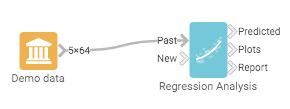A typical workflow with Regression

Omniscope enables users to provide more complex, non-linear, multivariate, robust estimations with intuitive graphical results. By default, the analytics block automatically chooses between a linear, logistic or count (Poisson) regression, however this selection can also be specified by the user.

Typical Use Case: Regression is best used when the user wants to estimate the effect of a change in the values of a series of variables upon a single variable of interest. A standard regression table provides coefficient estimates and p-values for more technical users, this is given in the "Report" output. The "Plots" output shows how the "Field to Predict" changes with each of the "Fields to Use", or in more technical terms how the dependent variable changes with each of the independent terms. "Prediction" estimates the effect of an updated set of "Fields to Use" upon the "Field to Predict".

This type of analysis is best used when the data is cross-sectional, i.e. the time period of the observation is not considered in the analysis.

## Contents

Generalised Linear Regression

## Case Study for a Generalised Linear Regression

Regression is frequently used in the Insurance industry. Here the insurer can use its data on past customers to estimate the likelihood of new claims based upon each of the claimant's attributes.Insurance data used in this case study

Here we have a data set containing attributes of holders of car insurance, this is available in the Demo Data block entitled "Car Insurance Claims". Each row represents a particular subset of holders, so the first row live in “District 1”, drive a car with an engine smaller than 1 litre, and are below 25. This represents 197 holders, and this group made 38 claims.Completed workflow with diagnostic message

Suppose we wanted to find out how District, Group, Age and Holders affected Claims. When the block is run the block automatically chooses to use a Poisson regression (optimal for this data set). By selecting the Regression Report we get the following results:Typical regression report

This provides a set of results that those familiar with regression would recognise, and the block automatically creates dummy variables for the categorical fields. Quickly plotting the Estimates and Variable Effect in Omniscope makes it apparent to the user that the most important factors to the number of claims made is AGE in the subgroup. In this case it is AGE, as those who are above 35 years old are more likely to make claims.Plotting the estimates and the variable effect

By choosing the Plots output we can more clearly see how the number of claims varies with the age group.How age varies with number of claims.

Suppose there are a new set of potential insurance holders and the firm wants to predict how many claimants there will be in each group. As a quick example we will take a Custom R block to take a random sample of the data, providing a training set and a set to perform a prediction on. This can serve as a measure of accuracy for the model.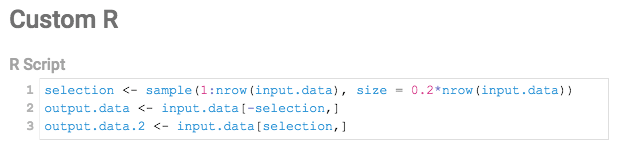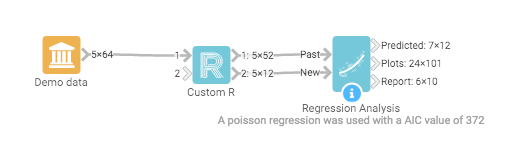A simple R script to take two Random samples of the data with the associated workflow.

The results from the prediction are very close to the actual data. This is indicative of the model effectiveness upon such data sets.Prediction results

## Case Study for Multivariate Adaptive Regression Splines

Multivariate Adaptive Regression Splines can be used if your data doesn't follow a straight linear trend. Sometimes data follows a linear trend up to a certain point and then changes its rate of ascend or descend from that point onward. In such a case using a linear regression would not provide the best fit to the data. Instead, a piecewise linear regression for both sides of the point of change might provide an improved fit.

# Input Data

We have here the bond prices demo data from which we will try to establish a relationship between coupon rate and issue date of the bond. Because issue date is a date, we would not expect for the coupon rate to have a straight linear trend. Instead, we would expect to see fluctuations, peaks and downs over time. We would also not expect to see a perfect perdiodic relationship (so that we could use a timeseries analysis). In order to model this kind of relationship, we need to find piecewise linear (or curved) relationships, as such we use multicariate adaptive regression splines.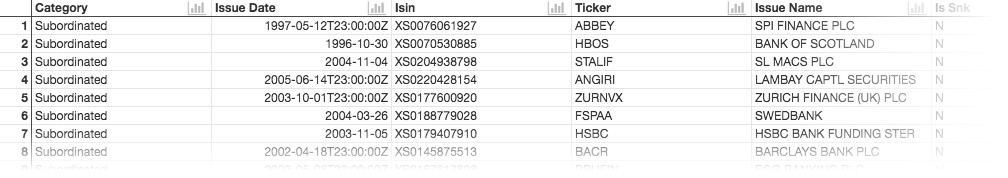The Bond Procves Demo Data Set

# Workflow

We create a multivariate adaptive regression spline workflow by connecting it with the Demo Data block and selecting the Bond Prices Demo Data Set.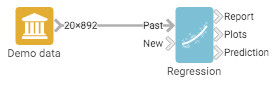Multivariate Adaptive Regression Splines example workflow

# Options

Once the block is connected, we can start setting it up.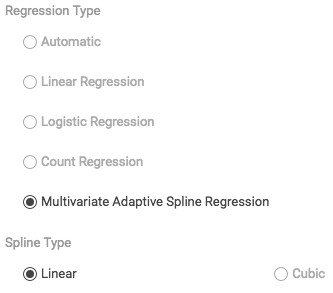Options

Regression Type: Here, we need to select "Multivariate Adaptive Spline Regression".

Spline Type: Select here whether your model should contain of piecewise straight lines (Linear) or instead use slightly curved segments (Cubic).These options give you a finer control over the actual output.

Robust Estimation: Check this checkbox if your data contains many outliers. With Robust Estimation, those outliers will be ignored which might improve your regression model.

Interactions: If this checkbox is checked, interaction terms between regressor variables will be included in the model.

Prediction with full posterior: During the course of the model building, the algorithm will create a posterior distribution of possible models. If this checkbox is unchecked, then the single best model will be used as a model for prediction. If the checkbox is checked instead, a weighted subset of all models will be used, which increases robustness and model precision with some additional computational cost.

Number of models from posterior: If "Prediction with full posterior" is checked, you can set here the number of models which will actually be used from the posterior distribution. Setting this number very high will result in additional computational cost.

# Output

If we plot Issue Data versus Coupon. we see a series of linear lines going through the data. As expected, throughout the years we see variations in the ascend and descend of the coupon rate. There is a strong peak at the beginning of the nineties and since then two downward trends with a slight increase at the millenium.Example graph